## Example Questions

### Example Question #11 : General Fractions

To make 48 cookies you need 1 cup white sugar, 1 cup packed brown sugar and 3 cups of flour.  You want to make 12 cookies, so you adjust the ingredients accordingly.  After the adjustment, how many total cups of dry ingredients do you have?

1 1/2 c

3/4 c

1 1/4 c

1 3/4 c

1/4 c

1 1/4 c

Explanation:

Going from 48 cookies to 12 cookies is a scaling factor of 1/4, so all the ingredients get multiplied by 1/4.  In order to make 12 cookies you will need 1/4 c white sugar, 1/4 c packed brown sugar, and 3/4 c flour.  Added all together you get 5/4 or 1 1/4 c.

### Example Question #2 : Mixed / Improper Fractions

What is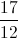written as a mixed number?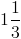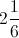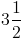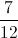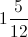Explanation:

How many times does 12 go into 17?  Once with a remainder of 5.

Sobecomes### Example Question #3 : Mixed / Improper Fractions

Which of the following is the mixed fraction equivalent to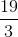?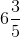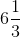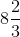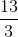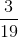Explanation:

To begin, notice that using your calculator, you can find: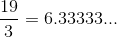Now, the closest even multiple ofthat is less thanis.  Therefore, you know that your number is: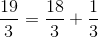This is the same as: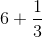, or simply,.  This is your mixed fraction.

### Example Question #1 : How To Find Out A Mixed Fraction From An Improper Fraction

Which of the following is equivalent to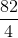?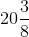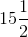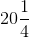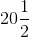Explanation:

Although there are many ways to convert improper fractions into mixed fractions, the easiest way is to use your calculator to your advantage.  Begin by dividing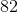by.  This gives you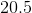. Therefore, you can eliminate all the options that have do not havefor their first portion. Next, multiplyby the denominator (), and get.  This means that you haveand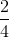, or.  Thus, your answer is.

### Example Question #1 : Mixed / Improper Fractions

Convert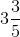to an improper fraction.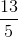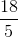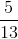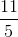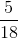Explanation:

The denominator will stay the same.  The new numerator of the improper fraction can be determined by multiplying the denominator with the whole number coefficient, and then adding the numerator.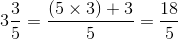### Example Question #6 : Mixed / Improper Fractions

Change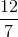into a mixed fraction.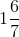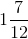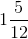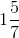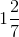Explanation:

Divide 12 by 7. This means that seven goes into twelve one time. One times seven gives you seven. From here we subtract seven from twelve which give us our remainder of five.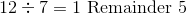The remainder of five is a fraction of seven.

Write the mixed fraction.### Example Question #11 : Mixed / Improper Fractions

Which of the following mixed numbers is equal to the improper fraction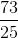?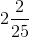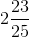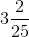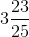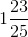Explanation:

To determine the coefficient of the mixed number, divide 73 by 25.  There will be a remainder since 73 does not go perfectly into 25.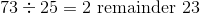There are two whole units and 23 of 25 units.  Rewrite this into a mixed fraction.

The correct answer is:### Example Question #1 : How To Find Out A Mixed Fraction From An Improper Fraction

Reduce the fraction below to its most simplest form.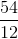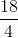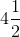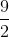Explanation:

In order to reduce a fraction, you need to divide each number by the same number. It is helpful to find the greatest common factor and divide by that. You can also divide it by a common factor a few times if you cannot determine the greatest common factor.

In this fraction, the GCF is.

If you divide both numbers by the GCF you get.

You then need to make the improper fraction into a mixed number. Determine how many timesgoes into. It will go in 4 times with 1 left over.

Therefore, the answer is.